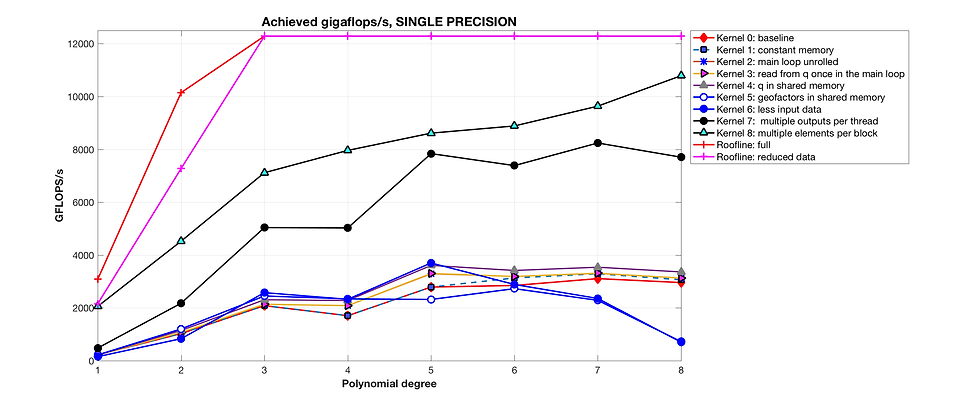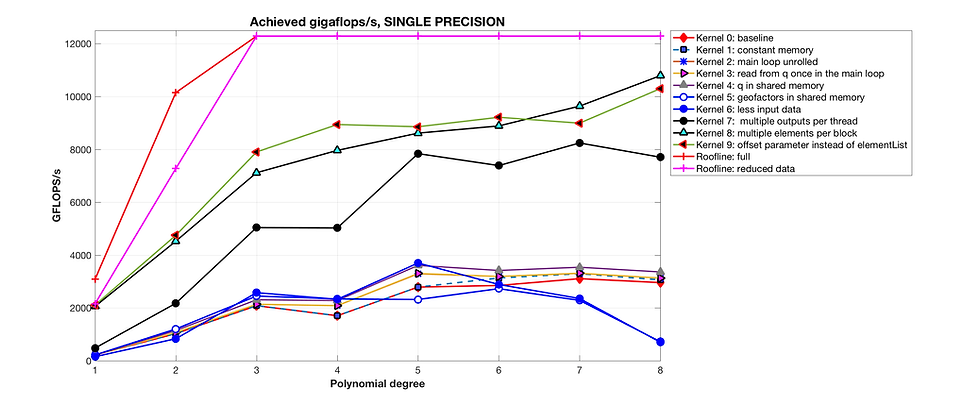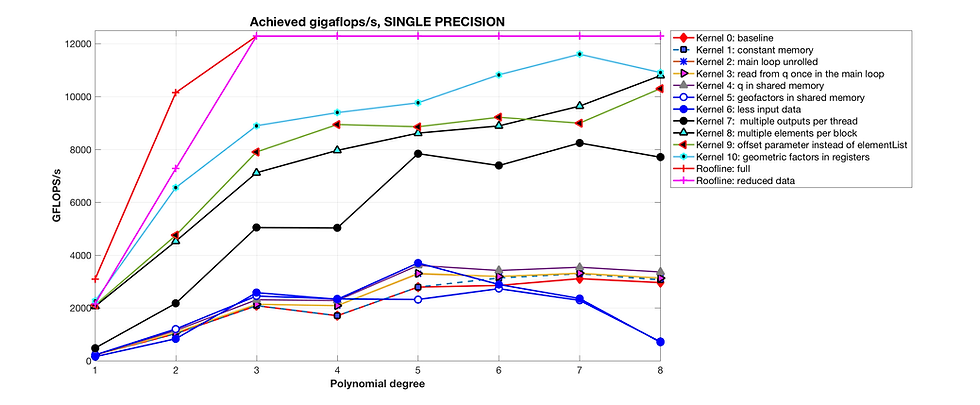top of page

Our Recent Posts

Archive

Tags

No tags yet.

# Finite Element Stiffness Matrix Action: monolithic kernel optimization on Titan VIn this post we demonstrate how to optimize the performance of a specific finite element operation expressed as a GPU kernel.

One might ask: why are we doing this? Why do we care so much about optimizing performance? After carefully reading this post, it should be clear that there is a substantial difference in performance between basic GPU code and highly optimized GPU code.

As our example we use a simple, yet demanding (high arithmetic intensity) matrix-vector kernel coming from FEM. The piece of code in question is executed multiple times per time step for time dependent flow calculations that might require hundreds of thousands of time steps, hence we would like to make it as fast as possible.

Note: We use OCCA in the code listings but the same techniques can be equally well applied directly in CUDA and OpenCL.

Discretization parameters: The code is executed for polynomial degrees N=1,2, ..., 8, using a mesh with E = 320000 affine tetrahedral elements. The number of nodes per element is given by the formula: Np = (N+1)(N+2)(N+3)/6. Note that Np grows with the third power of polynomial degree.

Input variables:

1. field variable q, an array of size [ Np x E. ]

2. nine operator matrices (Sss, Ssr, Sst, Srs, Srr, Srt, Sts, Str, Stt), each with dimension [ Np x Np ],

3. mass matrix, MM, dimension [ Np x Np ],

4. geometric factors with 7 constants per element, held in an array of size [ 7 x E ],

5. parameter lambda: one real value,

6. Integer-values elementList array: list of subset of elements indices, dimension [ E x 1 ].

The operator matrices are constructed relative to a reference element and are consequently the same for all the elements.

Output variable:

1. field variable Aq, an array of size [ Np x E ].

Initial kernel implementation: The "baseline" OCCA OKL kernel follows. Note that we store the operator matrices in column major form. This is important for coalescing as we perform matrix-vector multiplication with each thread executing a row/vector dot product.

Note: in the code the datafloat type is defined at runtime through the OCCA API as either float or double.

We start with some pen-and-paper (and calculator) analysis. In these codes p_Np is a compiler parameter defining the number of nodes per element.

Q: How many flops (per element) do we perform?

A: In this case: 20*p_Np^2 + 20*p_Np. Note that p_Np increases cubically with the polynomial degree and number of flops is proportional to the sixth power of the polynomial degree - exhibiting the dreaded curse of dimensionality.

This gives:

N | Number of flops per element

1 | 400

2 | 2200

3 | 8400

4 | 25200

5 | 63840

6 | 142800

7 | 290400

8 | 547800

Why this optimization is a challenge: as we see above, the kernel is characterized by high arithmetic intensity, i.e. we perform a lot of floating point operations. In addition, for example for N=8, the operator matrices together with the mass matrix consume 2.178 MB, which greatly exceeds the available shared memory and L1 cache on each SM.

The performance of the Ref0 kernel in times of achieved GFLOPS/s is:The empirical roofline consists of the sloping region where the kernel is memory bound because the arithmetic intensity (flops/byte accessed in device memory) is too low to fully occupy all of the floating point units. The horizontal part of the roofline is just the maximum floating point performance by manufacturer spec.

Optimaztion #1: We start by declaring all pointers to arrays that are only used for input as "const"

This did not yield any evident benefit in computational throughput:Optimzation #2: In the next step of the optimization process, we unroll the main innermost loop. Unrolling instructs the compiler to copy and paste the body of the loop and remove the conditional loop statement and the branch used to determine if the loop should continue. This sometimes helps the compiler to identify instruction pipelining opportunities, also known as Instruction Level Parallelism or ILP.

This did not yield any obvious benefit either:Optimization #3: We note that q[k+element*p_Np] is read multiple times in the loop. We can read it once to register and reuse. Thus, we code another kernel:

Sadly, we see no improvement. The compiler again is already likely ahead of us on this one.Optimization #4: Next, we preload an element worth of node data from the q array into shared memory:

However, as these calculations were benchmarked on the Titan V it is likely that in most cases the L1 cache was already being used to cache the solution data from q. We again see that the performance was barely impacted by this optimization:Optimization #5: In our next optimization we prefetch the element geometric factors to shared memory as well:

However, not only we did not make the code faster - we actually made it slower! A possible reason for this is that geometric factors are the same for the entire element so they are already in L1 cache and can be retrieved fast. Or the code used to fetch the geometric factors uses a poorly conceived access pattern.Optimization #6: Next we eliminate some of the input data by observing that we can exploit the symmetry of the 9 reference stiffness matrices and that Srt=Str^T, Srs=Ssr^T, Sst=Sts^T. This requires modifying the operator setup. We assume that SrtT actually contains SrtT + StrT and that SstT actually contains SstT + StsT.

The floating point performance does not improve, but the good news is that we have reduced the operation count from 20 p_Np flops to 14 p_Np flops per node. Thus the runtime has actually decreased by 30%.

Note: the roofline is modified because we move less data.Optimization #7: Our next optimization employs multiple outputs per thread. This is an attempt to change the ratio of floating point operations per access to L1+shared. In all the above kernels this ratio was fixed at approximately one cache access per two floating point operations. Thus the kernels are limited to approximately 13.3TB/s/(4B/2flops) = 6.6TFLOPS/s and in practice we see even lower performance. The adjusted bound is shown as the dashed red line in this figure:As seen below each thread is responsible for computing the output for the same index node but in p_Ne elements. It can thus load entries from the operator matrices into register and use them p_Ne times:

FINALLY! We see true improvement. Note that we optimize the choice of p_Ne by computational tuning.Optimization #8: In this optimization we use p_Nb elements per thread-block (times p_Ne elements for multiple outputs). The idea is to increase the number of threads per thread-block and (particularly at low-order) populate the thread-block with a number of threads closer to a multiple of the SM SIMD width:

This brings true improvement. One should note that we tune to co-optimally choose Ne and Nb for every polynomial degree N and the results show the performance of Kernel 8 with the best parameters.Optimization #9: We note that in Ref8 we loaded the geometric factors into shared memory in a non-coalesced way. Hence, we change it in Ref9. We also replace elementList by elementOffset which is just one integer assuming that the elements have been reordered so that they can be processed in continuous chunks:

This brings us small yet noticeable improvement at most orders:Optimization #10: instead of loading geometric factors to the shared memory, we fetch them just in time at the end of the kernel. We also rely on elementOffset as in Kernel 9 and here is the code in full:

We obtain a further improvement:One might think that the first 6 kernels were simply waste of time and effort. However, in order to get good speedup in our last kernel, we explored each and every optimization.

The graph below shows the time ratio (best kernel time divided by worst kernel time) for each polynomial degree.FP64: we switched datafloat to double and reran the above experiments. We see that although most of the kernels do not hit the roofline, the best kernels are achieving a reasonable fraction of peak attainable performance.The difference between the best and worst kernels at each polynomial degree is also quite startling. Some optimizations were bad, causing poor memory access of geometric factors for instance, and this has boosted the largest differences.Conclusions: It is clear that there is still a gap between the roofline an the achieved performance. The gap is more pronounced for mid-range degrees. We hypothesize that this gap results from L1 and L2 cache misses. For example, for DP and N=8, the Srr, Srs, ..., matrices alone take more than 4MB, which overflows even L2 cache.

Note: that we have used manufacturer peak floating point performance for the roofline plateaus. For an empirical estimate of the actual achievable peak performance on the Titan V see the earlier blog entry discussing the results from occaBench. Using the empirical peak suggest that the above results are close to best achievable performance for this kernel.

The performance of DP version is also surprisingly good. This indicates that NVIDIA substantially improved the DP performance for its flagship gaming video card.

We will explore an alternative approach using cuBLAS to do the matrix-matrix multiplication heavy lifting in a subsequent blog post.

Single post: Blog_Single_Post_Widget
bottom of page